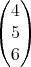Though Archimedes is designed as a program for synthetic geometry it can be used to visualize analytical geometry as well.

##### 4.7.1 Enter line in parametric formIn the figure above the line=+ swas entered. Archimedes generates the line, the position-vector and the direction-vector for the line.

You can enter expressions (e. g. sliders) as well as numbers in this dialogue. You must make this known to the program by checking the corresponding checkbox.

If you do not want the supporting point and the direction vector to be shown you can adjust that with the checkbox ’Show auxiliary objects’.

##### 4.7.2 Plane

Here equations for planes in parametric form, normal form and general form can be entered.In the above figure, the plane throughwith normalwas entered. Archimedes generates the plane, the position vector and the normal vector.

##### 4.7.3 Sphere

The equation for a sphere can be entered here by giving its midpoint and radius.

##### 4.7.4 Parametric curve

The equation for a parametric curve can be entered here, i.e.=for a helix.

##### 4.7.5 Parametric surface

This dialogue lets you enter the equation for a parametric surface. The ’Klein bottle’ from the examples-folder, subfolder ’functions’ can be generated as a parametric surface.

##### 4.7.6 Co-ordinate axes

Choosing this menu generates the co-ordinate axes as lines. Additionaly the origin and the unit-vectors are pasted into the construction.

##### 4.7.7 Co-ordinate planes

The xy-plane, yz-plane and xz-plane are pasted into the construction. A grid-texture is displayed on the planes. As long as you do not change the plane’s size, the meshes of the grid are one length unit long.

An example of using Archimedes for solving a typical high school exercise can be found in chapter ’Commented examples’, subsection analytical geometry.